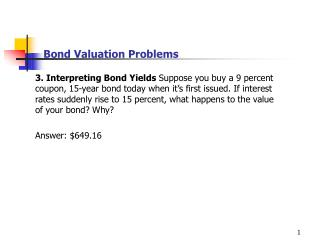DownloadDownload PresentationBond Valuation Problems

# Bond Valuation Problems

Download Presentation## Bond Valuation Problems

- - - - - - - - - - - - - - - - - - - - - - - - - - - E N D - - - - - - - - - - - - - - - - - - - - - - - - - - -
##### Presentation Transcript

1. Bond Valuation Problems • 3. Interpreting Bond Yields Suppose you buy a 9 percent coupon, 15-year bond today when it’s first issued. If interest rates suddenly rise to 15 percent, what happens to the value of your bond? Why? • Answer: \$649.16

2. Bond Valuation Problems • 5. Bond Yields N&N Co. has 11 percent coupon bonds on the • market with 8 years left to maturity. The bonds make annual • payments. If the bond currently sells for \$1,110.70 what is its YTM? • Answer: 9%

3. Bond Valuation Problems • 6. Coupon rates Merton Enterprises has bonds on the market • making annual payments, with 14 years to maturity, and selling for • \$950. At this price, the bonds yield 7.5 percent. What must the • coupon rate be on Merton’s bonds? • Answer: 6.911%

4. Bond Valuation Problems • 22. Interest Rate Risk Bond J is a 4 percent coupon bond. Bond • K is a 10 percent coupon bond. Both bonds have 10 years to • maturity, make semi-annual payments and have a YTM of 9 • percent. If interest rates suddenly rise by 2 percent, what is the • percentage price change of these bonds? What if rates suddenly • fall by 2 percent instead? What does this problem tell you about • the interest rate risk of lower-coupon bonds?

5. Bond Valuation Problems • 23. Bond Yields BrainDrain Software has 12 percent coupon • bonds on the market with 9 years to maturity. The bonds make • semiannual payments and currently sell for 125.32 percent of par. • What is the current yield on BrainDrain bonds? The YTM? The • effective annual yield? • Answer: CY=9.58% YTM=8% EAR=8.16%

6. Example. Decomposition of total return • when YTM at the end of a period is same as YTM at the beginning • of the period then • total realized return=YTM at the beginning of the period = • capital gains yield + interest income • for instance: • coupon rate=9% annual coupon N=10 years P=\$882.22 • P10=882.22= 90 PVIFAk,10 + 1000PVIFk,10 • k=11%=YTM at the beginning of the period

7. Example. Decomposition of total return • after 1 year if YTM is still 11% • P9= 90 PVIFA11%,9 + 1000PVIF11%,9=\$889.26 • total realized return=0.798%+10.202% =11% =YTM at the • beginning of the period

8. Example. Decomposition of total return • if YTM rises to 13% at the end of the period • P9=\$794.73 • total realized return=-9.917%+10.202% =0.285%  YTM at the • beginning of the period • Note: To be able to calculate realized return for more than one • period we need to make assumptions about reinvestment rates.

9. Bond Valuation Problems • 40. Holding Period Yield The YTM on a bond is the interest rate you • earn on your investment if interest rates don’t change. If you actually sell the bond • before it matures, your realized return is known as the holding period yield (HPY). • Suppose you buy a 10 percent coupon bond making annual payments today for • \$1,064.18. The bond has 10 years to maturity. What rate of return do you expect • on your investment? • Two years from now, the YTM on your bond has declined by 3 percent, and you • decide to sell. What price will your bond sell for? What is the HPY on your • investment assuming that you reinvest coupons at HPY? Compare this yield to the • YTM when you first bought the bond. Why are they different? • Answer: • 1,064.18=100 PVIFA10,k + 1,000 PVIF10,k • YTM=9% • b. New YTM=9%-3%=6% • \$1,248.39=100 PVIFA8,6% + 1,000 PVIF8,6% • 1,064.18=100 PVIFA2,HPY + 1,248.39 PVIF2,HPY • HPY=17.361% note this assumes you could reinvest \$100 coupon at 17.361%